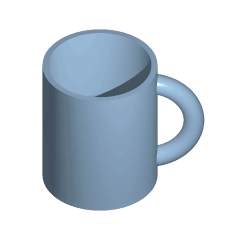## OT: Möbius strip [NCA / SHAM]

Hi Nastia,

❝ Hey, how did you know that I've got a model of it?I didn’t know but I’m not surprised.

❝ Unfortunatelly I am not good at topology…

cup → bagel → cup → …Welcome to the club. The worst is knot theory – I have books about it. Headaches guaranteed.
For sure you know what happens if we cut a Möbius strip once. Do you know what happens if we cut it twice? Makes a great party joke.

❝ But I know the answers for the questions what do we need to drill a square hole…

Punch, OK. But drill?

❝ … and also what profile one should use to ride a bike with square wheelsSo do I.

https://www.r-bloggers.com/topological-tomfoolery-in-r-plotting-a-mobius-strip
library(rgl) library(plot3D) ############### # Möbius band # ############### R <- 5 u <- seq(0, 2 * pi, length.out = 100) v <- seq(-1, 1, length.out = 100) m <- mesh(u, v) u <- m$x v <- m$y x <- (R + v/2 * cos(u /2)) * cos(u) y <- (R + v/2 * cos(u /2)) * sin(u) z <- v/2 * sin(u / 2)  invisible(open3d()) bg3d(color = "#F3F3F3") surface3d(x, y, z, color= "#87CEFA") title3d(main = "Moebius band") # Rotate with mouse-left, wheel to zoom. ################ # Klein bottle # ################ u <- seq(0, pi, length.out = 100) v <- seq(0, 2 * pi, length.out = 100) m <- mesh(u, v) u <- m$x v <- m$y x <- (-2 / 15) * cos(u) * (3 * cos(v) - 30 * sin(u) + 90 * cos(u)^4 * sin(u) -       60 * cos(u)^6 * sin(u) + 5 * cos(u) * cos(v) * sin(u)) y <- (-1 / 15) * sin(u) * (3 * cos(v) - 3 * cos(u)^2 * cos(v) - 48 *       cos(u)^4 * cos(v) + 48 * cos(u)^6 * cos(v) - 60 * sin(u) + 5 * cos(u) *       cos(v) * sin(u) - 5 * cos(u)^3 * cos(v) * sin(u) - 80 * cos(u)^5 *       cos(v) * sin(u) + 80 * cos(u)^7 * cos(v) * sin(u)) z <- (+2 / 15) * (3 + 5 * cos(u) * sin(u)) * sin(v) invisible(open3d()) bg3d(color = "#F3F3F3") surface3d(x, y, z, color= "#87CEFA", alpha = 0.5) title3d(main = "Klein bottle")

Dif-tor heh smusma 🖖🏼 Довге життя Україна!Helmut SchützThe quality of responses received is directly proportional to the quality of the question asked. 🚮
Science QuotesIng. Helmut Schütz